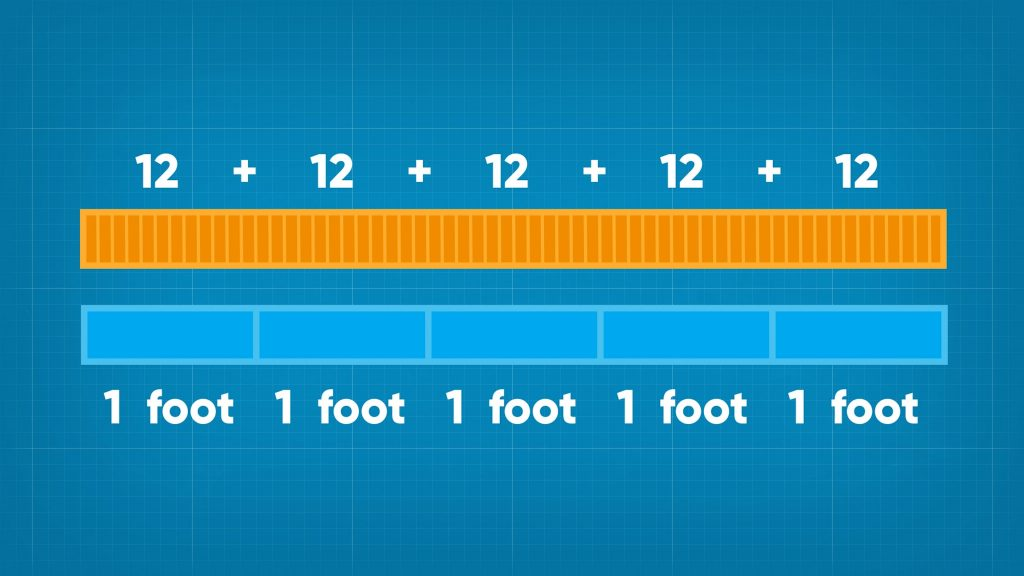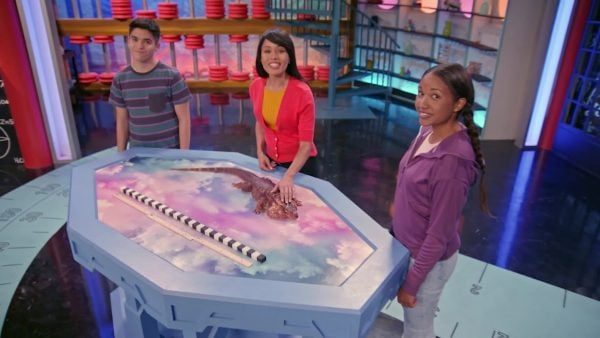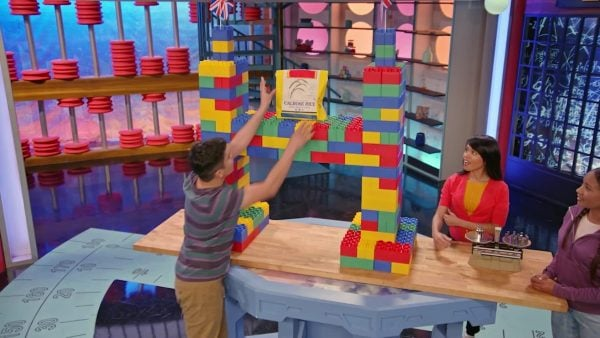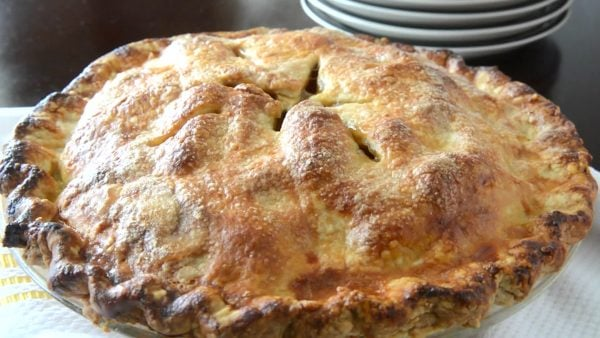Read about Converting Units of Measurement | for kids Grade 3, 4, & 5
1%
It was processed successfully!

# Read How to Convert Units of MeasurementWHAT IS CONVERTING UNITS OF MEASUREMENT?

You can convert between units to solve problems involving measurement.

To better understand converting units of measurement…

WHAT IS CONVERTING UNITS OF MEASUREMENT?. You can convert between units to solve problems involving measurement.  To better understand converting units of measurement…

## LET’S BREAK IT DOWN!

### The Imperial System of ft and in.: IguanasConverting units of length can help you determine if your iguana will fit inside the tank when he is fully-grown. Suppose April has a tank that is 36 inches long. She wants to know if the iguana will fit in the tank when he grows to 6 feet long. We can figure this out by finding how many inches are in 6 feet. Each foot is 12 inches. So, in 6 feet there are 12 + 12 + 12 + 12 + 12 + 12 = 72 inches. Repeated addition is multiplication, so we could also multiply 6 × 12 = 72 inches. We get the same answer. The tank is 36 inches long and the iguana will be 72 inches long, so the tank will not be big enough. Now you try. Suppose Marcos gets a spiney-tailed iguana that only grows to about 36 inches. Will a tank that is 4 feet long be big enough for his iguana?

The Imperial System of ft and in.: Iguanas Converting units of length can help you determine if your iguana will fit inside the tank when he is fully-grown. Suppose April has a tank that is 36 inches long. She wants to know if the iguana will fit in the tank when he grows to 6 feet long. We can figure this out by finding how many inches are in 6 feet. Each foot is 12 inches. So, in 6 feet there are 12 + 12 + 12 + 12 + 12 + 12 = 72 inches. Repeated addition is multiplication, so we could also multiply 6 × 12 = 72 inches. We get the same answer. The tank is 36 inches long and the iguana will be 72 inches long, so the tank will not be big enough. Now you try. Suppose Marcos gets a spiney-tailed iguana that only grows to about 36 inches. Will a tank that is 4 feet long be big enough for his iguana?

### Imperial Weights: Bridges!Converting units of weight can help you decide how much weight a bridge can hold. Suppose Marcos made a bridge from blocks that can hold a rock that weighs 32 ounces. He wants to know how many pounds the bridge can hold. There are 16 ounces in 1 pound. We can break 32 into groups of 16. 16 + 16 = 32. There are 2 groups of 16 in 32. Each group is 1 pound, so 32 ounces is the same as 2 pounds. Making equal groups of 16 is the same as dividing. We could divide 32 by 16. We get the same answer, 2. His bridge can hold 2 pounds. Now you try. Suppose Marcos builds another bridge that can hold a bag of flour that weighs 80 ounces. How many pounds can the bridge hold?

Imperial Weights: Bridges! Converting units of weight can help you decide how much weight a bridge can hold. Suppose Marcos made a bridge from blocks that can hold a rock that weighs 32 ounces. He wants to know how many pounds the bridge can hold. There are 16 ounces in 1 pound. We can break 32 into groups of 16. 16 + 16 = 32. There are 2 groups of 16 in 32. Each group is 1 pound, so 32 ounces is the same as 2 pounds. Making equal groups of 16 is the same as dividing. We could divide 32 by 16. We get the same answer, 2. His bridge can hold 2 pounds. Now you try. Suppose Marcos builds another bridge that can hold a bag of flour that weighs 80 ounces. How many pounds can the bridge hold?

### Metric Distance of cm, m, and km: Bike RidesConverting metric units can help you adjust your bike seat to the right height. Suppose the manual for April’s bike says that the height of her bike seat should be 1.24 meters. She measured the height of her seat and found that it is 112 centimeters high. There are 100 centimeters in 1 meter. We can multiply 1.24 meters by 100 to find how many centimeters high the seat should be. When we multiply by 10, we can just move the decimal point one place to the right. Multiplying by 100 is the same as multiplying by 10 twice. So, we can move the decimal point 2 places to the right. 1.24 × 100 = 124. This means that the height of her seat should be 124 centimeters. April’s seat is 112 centimeters high, so she should raise the seat. Now you try. Suppose the manual for Marcos’s bike says that his seat should be 1.37 meters high. He measured the height and found that it is 147 centimeters. Does he need to adjust the seat up, down, or not at all?

Metric Distance of cm, m, and km: Bike Rides Converting metric units can help you adjust your bike seat to the right height. Suppose the manual for April’s bike says that the height of her bike seat should be 1.24 meters. She measured the height of her seat and found that it is 112 centimeters high. There are 100 centimeters in 1 meter. We can multiply 1.24 meters by 100 to find how many centimeters high the seat should be. When we multiply by 10, we can just move the decimal point one place to the right. Multiplying by 100 is the same as multiplying by 10 twice. So, we can move the decimal point 2 places to the right. 1.24 × 100 = 124. This means that the height of her seat should be 124 centimeters. April’s seat is 112 centimeters high, so she should raise the seat. Now you try. Suppose the manual for Marcos’s bike says that his seat should be 1.37 meters high. He measured the height and found that it is 147 centimeters. Does he need to adjust the seat up, down, or not at all?

### Pie/DivisionConverting metric units of mass can help you make an apple pie. Let’s say you need 500 grams of sugar for apple pie filling. You can weigh the sugar on a scale, but the scale only measures in kilograms. We can find how many kilograms of sugar you need for the pie so you can use the scale. There are 1,000 grams in 1 kilogram. We need to convert grams to kilograms, so we are converting from a smaller unit to a larger unit. We can divide. When we divide by powers of 10, we can move the decimal point to the left. Dividing by 1,000 is like dividing by 10 three times. So, we can move the decimal point 3 places to the left. 500 divided by 1,000 is 0.5. You need 0.5 kilogram of sugar. Now you try. Suppose you need 700 grams of flour for the pie crust. How many kilograms of flour do you need?

Pie/Division Converting metric units of mass can help you make an apple pie. Let’s say you need 500 grams of sugar for apple pie filling. You can weigh the sugar on a scale, but the scale only measures in kilograms. We can find how many kilograms of sugar you need for the pie so you can use the scale. There are 1,000 grams in 1 kilogram. We need to convert grams to kilograms, so we are converting from a smaller unit to a larger unit. We can divide. When we divide by powers of 10, we can move the decimal point to the left. Dividing by 1,000 is like dividing by 10 three times. So, we can move the decimal point 3 places to the left. 500 divided by 1,000 is 0.5. You need 0.5 kilogram of sugar. Now you try. Suppose you need 700 grams of flour for the pie crust. How many kilograms of flour do you need?

## CONVERT UNITS OF MEASUREMENT VOCABULARY

Unit
A quantity used as the standard to tell how much makes up ‘1’ of the measurement. For example, units of length include inches, feet, centimeters, and meters.
Unit conversion
The process of changing from one unit of measurement to another.
US Customary system
A system of measurements used to measure length, weight, capacity, and temperature.
Metric system
A system of measurement that uses meters to measure length and distance, liters to measure capacity (volume), and grams to measure weight (mass).
Convert (measurement)
To change from one unit to another.
Length
A measurement of the distance between two endpoints.
A measurement of how heavy an object is.

## CONVERT UNITS OF MEASUREMENT DISCUSSION QUESTIONS

### How do you convert 7 feet to inches? How many inches are in 7 feet?

Convert 7 feet to inches by multiplying 7 by 12. There are 84 inches in 7 feet.

### How did you know to multiply when converting from feet to inches?

I converted from larger units to smaller units.

### How do you convert 80 ounces to pounds? Explain how you know.

Divide 80 by 16 because I start with a smaller unit and convert to a larger unit. There are 16 ounces in a pound.

### Which is longer, 1.2 meters or 121 centimeters? How do you know?

121 centimeters is longer; 1.2 meters is 120 centimeters. 121 is greater than 120.

### How can you remember to use the number 1,000 to convert grams to kilograms and kilograms to grams?

‘Kilo’ means 1,000. So, a kilogram is 1,000 grams. To convert kilograms to grams, multiply by 1,000. To convert grams to kilograms, divide by 1,000.
X

## Success

We’ve sent you an email with instructions how to reset your password.
Ok